# Percus Yevick

If one defines a class of diagrams by the linear combination (Eq. 5.18 Ref.1) (See G. Stell in Ref. 2)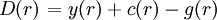$\left.D(r)\right. = y(r) + c(r) -g(r)$

one has the exact integral equation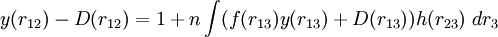$y(r_{12}) - D(r_{12}) = 1 + n \int (f(r_{13})y(r_{13})+D(r_{13})) h(r_{23})~dr_3$

The Percus-Yevick integral equation sets D(r)=0. Percus-Yevick (PY) proposed in 1958 Ref. 3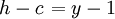$\left.h-c\right.=y-1$

The Percus-Yevick closure relation can be written as (Ref. 3 Eq. 61)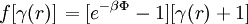$\left.f [ \gamma (r) ]\right. = [e^{-\beta \Phi} -1][\gamma (r) +1]$

or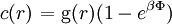$\left.c(r)\right.= {\rm g}(r)(1-e^{\beta \Phi})$

or (Eq. 10 in Ref. 4)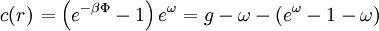$\left.c(r)\right.= \left( e^{-\beta \Phi } -1\right) e^{\omega}= g - \omega - (e^{\omega} -1 -\omega)$

or (Eq. 2 of Ref. 5)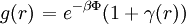$\left.g(r)\right. = e^{-\beta \Phi} (1+ \gamma(r))$

where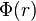$\Phi(r)$ is the intermolecular pair potential.

In terms of the bridge function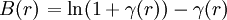$\left.B(r)\right.= \ln (1+\gamma(r) ) - \gamma(r)$

Note: the restriction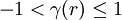$-1 < \gamma (r) \leq 1$ arising from the logarithmic term Ref. 6. A critical look at the PY was undertaken by Zhou and Stell in Ref. 7.